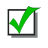Games
Problems
Go Pro!

# Slick Math

Reference > MathematicsCovering a partial checkerboard with 31 dominoes -- is it possible?Follow a set of rules to change the sequence of letters MI to MU.Does the series consisting of reciprocals of integers diverge or converge? The explanation is quite simple and elegant!Counterintuitive probability problems like the Monty Hall three door problem can be very frustrating. Here's a simple explanation.A geometry problem involving a rectangular prism, lengths of edges, surface area, and internal diagonals.How many prime numbers are there? This is a seemingly complex problem with a simple, elegant solution.Students open and close lockers -- how many are left open when they are finished?Like us on Facebook to get updates about new resources